### Estimating the Parameters in the Nonlinear Model

As an example of a nonlinear regression analysis, consider the following theoretical model of enzyme kinetics. The model relates the initial velocity of an enzymatic reaction to the substrate concentration.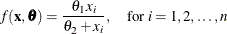whererepresents the amount of substrate for n trials and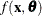is the velocity of the reaction. The vectorcontains the rate parameters. This model is known as the Michaelis-Menten model in biochemistry (Ratkowsky, 1990, p. 59). The model exists in many parameterizations. In the form shown here,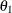is the maximum velocity of the reaction that is theoretically attainable. The parameter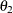is the substrate concentration at which the velocity is 50% of the maximum.

Suppose that you want to study the relationship between concentration and velocity for a particular enzyme/substrate pair. You record the reaction rate (velocity) observed at different substrate concentrations.

A SAS data set is created for this experiment in the following DATA step:

data Enzyme;
input Concentration Velocity @@;
datalines;
0.26 124.7   0.30 126.9
0.48 135.9   0.50 137.6
0.54 139.6   0.68 141.1
0.82 142.8   1.14 147.6
1.28 149.8   1.38 149.4
1.80 153.9   2.30 152.5
2.44 154.5   2.48 154.7
;


The SAS data set Enzyme contains the two variables Concentration (substrate concentration) and Velocity (reaction rate). The following statements fit the Michaelis-Menten model by nonlinear least squares:

proc nlin data=Enzyme method=marquardt hougaard;
parms theta1=155
theta2=0 to 0.07 by 0.01;
model Velocity = theta1*Concentration / (theta2 + Concentration);
run;


The DATA= option specifies that the SAS data set Enzyme be used in the analysis. The METHOD= option directs PROC NLIN to use the MARQUARDT iterative method. The HOUGAARD option requests that a skewness measure be calculated for the parameters.

The PARMS statement declares the parameters and specifies their initial values. Suppose that V represents the velocity and C represents the substrate concentration. In this example, the initial estimates listed in the PARMS statement forandare obtained as follows::

Because the model is a monotonic increasing function in C, and because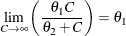you can take the largest observed value of the variable Velocity (154.7) as the initial value for the parameter Theta1. Thus, the PARMS statement specifies 155 as the initial value for Theta1, which is approximately equal to the maximum observed velocity.:

To obtain an initial value for the parameter, first rearrange the model equation to solve for: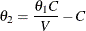By substituting the initial value of Theta1 forand taking each pair of observed values of Concentration and Velocity for C and V, respectively, you obtain a set of possible starting values for Theta2 ranging from about 0.01 to 0.07.

You can choose any value within this range as a starting value for Theta2, or you can direct PROC NLIN to perform a preliminary search for the best initial Theta2 value within that range of values. The PARMS statement specifies a range of values for Theta2, resulting in a search over the grid points from 0 to 0.07 in increments of 0.01.

The MODEL statement specifies the enzymatic reaction model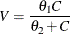in terms of the data set variables Velocity and Concentration and in terms of the parameters in the PARMS statement.

The results from this PROC NLIN invocation are displayed in the following figures.

PROC NLIN evaluates the model at each point on the specified grid for the Theta2 parameter. Figure 63.1 displays the calculations resulting from the grid search.

Figure 63.1: Nonlinear Least-Squares Grid Search

The NLIN Procedure
Dependent Variable Velocity

Grid Search
theta1 theta2 Sum of Squares
155.0 0 3075.4
155.0 0.0100 2074.1
155.0 0.0200 1310.3
155.0 0.0300 752.0
155.0 0.0400 371.9
155.0 0.0500 147.2
155.0 0.0600 58.1130
155.0 0.0700 87.9662

The parameter Theta1 is held constant at its specified initial value of 155, the grid is traversed, and the residual sum of squares is computed at each point. The best starting value is the point that corresponds to the smallest value of the residual sum of squares. The best set of starting values is obtained for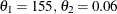(Figure 63.1). PROC NLIN uses this point from which to start the following, iterative phase of nonlinear least-squares estimation.

Figure 63.2 displays the iteration history. Note that the first entry in the Iterative Phase table echoes the starting values and the residual sum of squares for the best value combination in Figure 63.1. The subsequent rows of the table show the updates of the parameter estimates and the improvement (decrease) in the residual sum of squares. For this data-and-model combination, the first iteration yielded a large improvement in the sum of squares (from 58.113 to 19.7017). Further steps were necessary to improve the estimates in order to achieve the convergence criterion. The NLIN procedure by default determines convergence by using R, the relative offset measure of Bates and Watts (1981). Convergence is declared when this measure is less than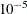—in this example, after three iterations.

Figure 63.2: Iteration History and Convergence Status

The NLIN Procedure
Dependent Variable Velocity
Method: Marquardt

Iterative Phase
Iter theta1 theta2 Sum of Squares
0 155.0 0.0600 58.1130
1 158.0 0.0736 19.7017
2 158.1 0.0741 19.6606
3 158.1 0.0741 19.6606

 NOTE: Convergence criterion met.

Figure 63.3: Estimation Summary

Estimation Summary
Method Marquardt
Iterations 3
R 5.861E-6
PPC(theta2) 8.569E-7
RPC(theta2) 0.000078
Object 2.902E-7
Objective 19.66059
Observations Used 14
Observations Missing 0

A summary of the estimation including several convergence measures (R, PPC, RPC, and Object) is displayed in Figure 63.3.

The R measure in Figure 63.3 is the relative offset convergence measure of Bates and Watts. A PPC value of 8.569E–7 indicates that the parameter Theta2 (which has the largest PPC value of the parameters) would change by that relative amount, if PROC NLIN were to take an additional iteration step. The RPC value indicates that Theta2 changed by 0.000078, relative to its value in the last iteration. These changes are measured before step length adjustments are made. The Object measure indicates that the objective function changed by 2.902E–7 in relative value from the last iteration.

Figure 63.4 displays the analysis of variance table for the model. The table displays the degrees of freedom, sums of squares, and mean squares along with the model F test.

Figure 63.4: Nonlinear Least-Squares Analysis of Variance

 Note: An intercept was not specified for this model.

Source DF Sum of Squares Mean Square F Value Approx
Pr > F
Model 2 290116 145058 88537.2 <.0001
Error 12 19.6606 1.6384
Uncorrected Total 14 290135

Figure 63.5: Parameter Estimates and Approximate 95% Confidence Intervals

Parameter Estimate Approx
Std Error
Approximate 95% Confidence
Limits
Skewness
theta1 158.1 0.6737 156.6 159.6 0.0152
theta2 0.0741 0.00313 0.0673 0.0809 0.0362

Figure 63.5 displays the estimates for each parameter, the associated asymptotic standard error, and the upper and lower values for the asymptotic 95% confidence interval. PROC NLIN also displays the asymptotic correlations between the estimated parameters (not shown).

The skewness measures of 0.0152 and 0.0362 indicate that the parameter estimators exhibit close-to-linear behavior and that their standard errors and confidence intervals can be safely used for inferences.

Thus, the estimated nonlinear model relating reaction velocity and substrate concentration can be written as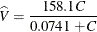where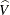represents the predicted velocity or rate of the reaction, and C represents the substrate concentration.